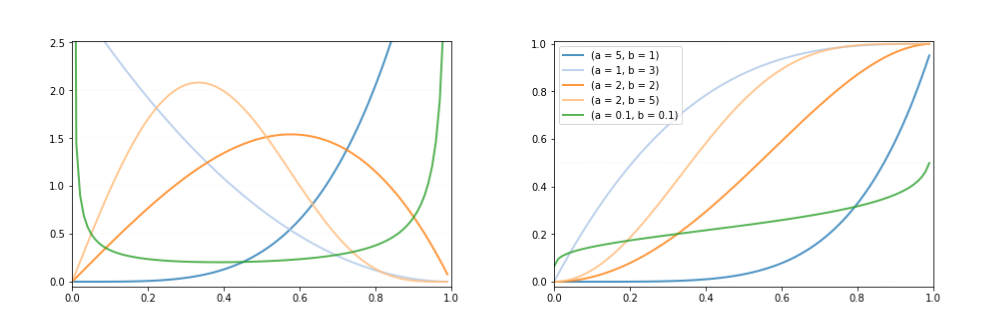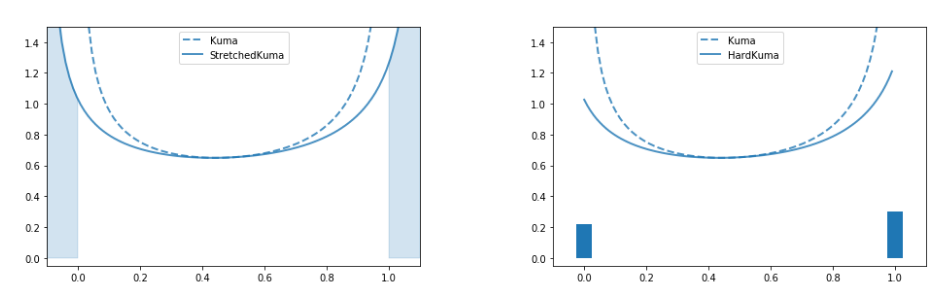# A note on Hard Kumaraswamy Distribution

This is an excerpt from my master thesis titled: “Semi-supervised morphological reinflection using rectified random variables”

In this story we describe the stretch-and-rectify principle applied to the Kumaraswamy distribution . This technique was proposed by Louizos et al., 2017  who rectified samples from a Gumbel-sigmoid distribution.The Kumaraswamy distribution pdf (left) and cdf (right) for various shape parameter values. The original version of this illustration is from Bastings et al. (2019).

## The Kumaraswamy distribution

The Kumaraswamy distribution (Kumaraswamy, 1980) is a doubly-bounded continuous probability distribution defined in the interval (0,1). Its shape is controlled by two parameters a∈R>0 and b∈R>0. If a=1 or b=1 or both, Kumaraswamy is equivalent to the Beta distribution. For equivalent settings of parameters, Kumaraswamy distribution closely mimics Beta distribution(but with a higher entropy). Its density function and is given below:

where a and b are shape parameters as previously mentioned. Its cumulative distribution function(cdf) can be derived as shown below:

## Sampling from Kumaraswamy distribution

We note that cumulative density function has the support [0,1]. Using the cumulative density function shown above, we can derive its inverse as follows:

where z∈[0,1] denotes the cumulative density function value. Therefore, to obtain a Kumaraswamy sample, we first sample from a uniform distribution with support [0,1] and transform it using the inverse cdf. With this formulation, we can reparameterize expectations as described in Nalisnick and Smyth, 2016 . The sampling procedure is shown below:

## Rectified Kumaraswamy distribution

Let k denote a base random variable sampled from Kuma(a,b). Its domain is the open interval(0,1). k is stretched to be defined in the open interval (l,r), where l <0,r >1 and we denote the stretched version ass. Its cumulative density function is shown below:

Finally, s is rectified to be defined in the domain [0,1] by passing it through a hard-sigmoid function, i.e., min(1,max(0,s)). We denote the rectified variable with h. Following Bastings et al.(2019)  we refer to the stretched and rectified distribution as Hard Kumaraswamy distribution. The probability of sampling exactly s= 0 is 0, since s is continuous in the interval (l, r), sampling any value exactly has a probability of 0. However, sampling h= 0 is equivalent to sampling any s∈(l,0]. Similarly sampling h= 1 is equivalent to sampling any s∈[1,r), i.e.Stretch and Rectify: we start from a Kuma(0.5, 0.5), and stretch its support to the interval (-0.1, 1.1), finally we collapse the mass below 0 to{0}and the mass above 1 to{1}. The original version of this illustration is from Bastings et al. (2019).

Figure above illustrates the process of stretch and rectify. The shaded region shows the probability of sampling h=0 (left) and h=1 (right). The rectified variable h has a distribution consisting of point mass at 0 and 1, and a stretched distribution truncated to (0,1),

where f(h) is the probability density function of H, δ(.) denotes the Dirac-delta function and T is the truncated distribution, and

where π₀ and π₁ denote the probability of sampling discrete outcomes,{0}and{1} respectively and π𝒸 denotes probability of sampling a continuous outcome. The truncated density fₜ(t) is introduced as fₛ(s) is properly normalized over (l,r). We can see that fₕ(h) has the following properties:

1. Support-consistency: It has support [0,1] and includes the discrete outcomes{0}and{1}.
2. Flexibility: It is possible to control the parameters of this distribution such that we can specify the probability of getting the outcomes{0}and{1}.
3. Differentiability: The distribution is differentiable almost everywhere with respect to its parameters to take advantage of off-the-shelf (stochastic) gradient ascent techniques.

# References

1. Bastings, J., Aziz, W., and Titov, I. (2019). Interpretable neural predictions with differentiable binary variables. arXiv preprint arXiv:1905.08160.
2. Louizos, C., Welling, M., and Kingma, D. P. (2017). Learning sparse neural networks throughl0 regularization.arXiv preprint arXiv:1712.01312.
3. Nalisnick, E. and Smyth, P. (2016). Stick-breaking variational autoencoders.arXiv preprintarXiv:1605.06197.

--

--